Technical Information Site of Power Supply Design

• Basic Knowledge
• AC/DC
• Isolated Flyback Converters: Performance Evaluation and Check Points
• Critical checkpoint: Measuring temperature and loss

2017.06.08 AC/DC

# Critical checkpoint: Measuring temperature and loss

Isolated Flyback Converters: Performance Evaluation and Check Points

In relation to evaluation of the performance of an isolated flyback converter, we begin a discussion of "Measuring temperature and loss", as a "Critical check point" other than device specifications that should be examined.

In this section, we explain methods for measuring and calculating temperatures and losses. The purpose is to confirm that the temperature Tj at the junction of the power supply IC does not exceed the absolute maximum rated temperature Tjmax even during maximum-temperature conditions in actual operation. The absolute maximum ratings must never be exceeded at any time, and therefore a state in which they are exceeded must not be allowed to occur. If they were exceeded, the device lifetime could be shortened dramatically, possibly resulting in breakdown. Apart from not exceeding the absolute maximum rated temperature, from the standpoint of reliability, this is also relevant for understanding the actual Tj and for derating.

In addition, losses must be checked, because losses = generation of heat. Seen from the standpoint of allowed losses, actual losses should be checked to ensure that they are at or below the allowed loss amount, but ultimately it all comes down to the Tj value. In relation to this, by understanding loss-related factors, in some cases losses can be ameliorated and heat generation can be reduced. Of course this also leads to improvement of efficiency.

【Conditions to check】
• Input voltage: Maximum
• Load current: Maximum
• Environment: Temperature condition upper limit
【Check points】
• Whether the Tj of the power supply IC exceeds the absolute maximum rated temperature
• Whether losses are less than the allowable losses indicated by the datasheet

Temperature Measurements

The power supply IC used in the example here has an internal power transistor, and so the junction temperature of the power supply IC is measured. In the case of a power supply IC with an external power transistor, the most important source of heat generation is the external power transistor, but there are instances in which the current to drive the power transistor gate of the controller IC is not small, and due care should be exercised.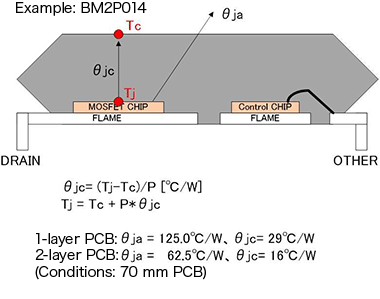It is necessary to know the junction temperature Tj, but IC chips are generally resin-sealed within a package, and so direct measurement is not possible. Hence Tj is calculated based on thermal resistance and the actual measured values of the ambient temperature Ta and the case (package) temperature Tc. The diagram on the right shows the relation between thermal resistance and temperature. The thermal resistance θja is the total thermal resistance from the junction (chip) to the ambience. Tj can be found from the following equations, which draw on the basic fact that Tj is due to Ta plus internal heat generation, and heat generation is the product of thermal resistance and electric power.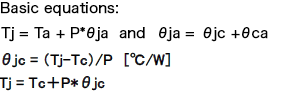Where the thermal resistance is concerned, the data supplied by the IC manufacturer is referenced. If it does not appear on a datasheet or the like, try asking the manufacturer directly.

Ta or Tc is thus actually measured, but measuring Ta is surprisingly difficult. Measurements of the ambient temperature may be performed using a thermocouple, but near the heat-generating body, the temperature is considerably different from a distant location. Moreover, in actual equipment there are the problems of under what conditions a measurement should be regarded as the Ta value, whether inside the housing, with air moving as a cooling fan spins, and so on. It is a difficult problem, although resolution relying on empirical rules is possible.

In contrast, measurement of Tc has become comparatively simple in recent years, with the spread of thermography, radiation thermometers, and other technologies. Of course, a thermocouple can also be used in measurements, but the thermocouple must be brought into contact with the package, and heat dissipation by the thermocouple occurs, so that accurate measurements are quite difficult. Never under any circumstances tape the end of a thermocouple to the top of a package to perform measurements! Considering the recent situation, it is probably best to use a contact-free thermometer that uses infrared rays.

Another question is where on the package the temperature should be measured to use as the Tc in these calculations. It is probably easy to understand when viewing the package diagram above, but Tc is different directly above the power transistor and above the control chip, which does not consume much power. In essence, the highest temperature value on the package surface is used. In this respect, thermography is convenient. It should also be noted that when a thermocouple is used, there are other, more detailed conditions, but in general, the temperature at the package center is measured.

Measurement of Losses

When data for the thermal resistance and Tc or Ta has been obtained, what is necessary for calculation using the above equation is P, that is the consumed power = (power) loss. The loss is obtained simply by computing voltage x current. For example, in an LDO linear regulator, the power being lost is (input voltage - output voltage) x (output current + self-current consumption). In the case of a switching regulator, power is chopped by switching, and so the average power consumption must be determined; this calculation is somewhat complicated. The basic calculation equation is shown below.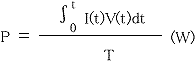In essence, the average power P is the value obtained by integrating the product of the current and voltage with respect to time, divided by the time T. This is applied to actual switching. As a general approach, a single period is assumed to have four states, which are off → on (T1 to T2), an in interval (T2 to T3), on → off (T3 to T4), and an off interval (T4 to T5). Calculations are performed for the four sections, as indicated below. An integral formula is used in actual calculation of the integral.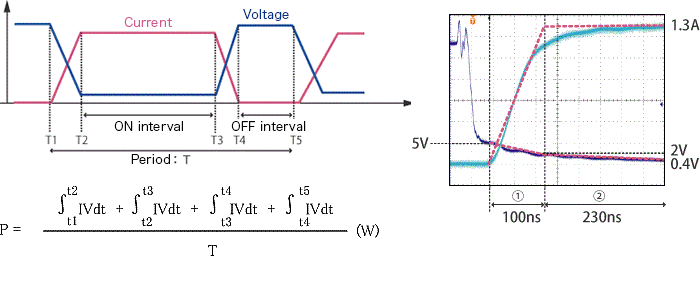In order to perform these calculations, the times of the above-described four intervals, and waveform data from which voltages and currents can be read off are necessary. In particular, it is necessary to acquire a waveform from which the relation between the rise/fall time during transitions and the voltage/current settling time can be acquired (see the waveform data on the upper right). The read-off values are substituted into the equation to perform the calculation.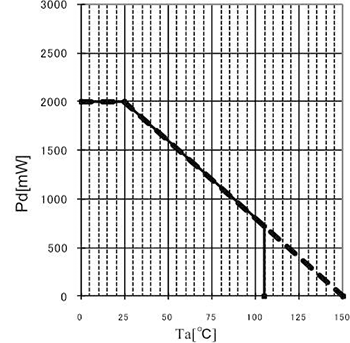Summary

The loss P (power consumption) determined by measurements and calculations is substituted into the above equation for Tj to determine Tj. The absolute maximum rated value for Tj is 150°C, and so the value obtained is confirmed to be equal to or below this value.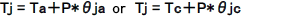The allowed loss is determined from the graph on the right (excerpted from the BM2P013 datasheet, when mounted on a 74.2 mm x 74.2 mm x 1.6 mm glass epoxy two-layer PCB). The IC has an operating temperature range with Ta = 105°C; we see that at that temperature, power consumption of approx. 750 mW can be allowed. In order to enable use as a power supply at the maximum operating temperature, the Ta near the IC is necessary. The minimum required condition for operation is that the losses be kept within the allowed loss range under all temperature conditions.

In this section, we have presented mainly a theoretical discussion, but the ROHM website provides actual methods and examples of calculation of temperatures and losses, and should by all means be consulted as well.

#### Key Points:

・Confirming that the junction temperature (Tj) of a device (chip) is within the absolute rated value is essential, for safe operation as well as to ensure reliability.

・Temperatures are determined by actual measurements and utilizing the concept of thermal resistance.

・Losses are determined by observing switching waveforms and using equations.

・Allowed losses are determined by consulting a graph on the datasheet, but ultimately are based on the value of Tj.

This website uses cookies.

By continuing to browse this website without changing your web-browser cookie settings, you are agreeing to our use of cookies.